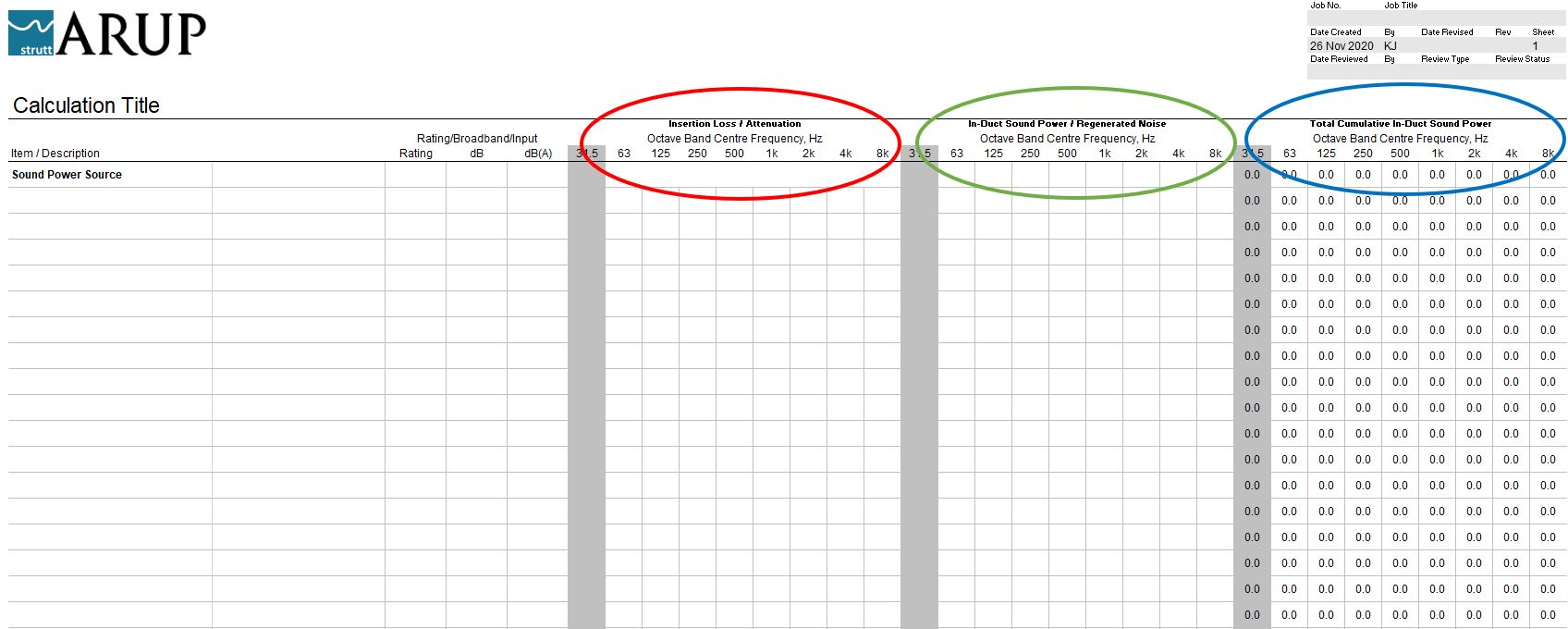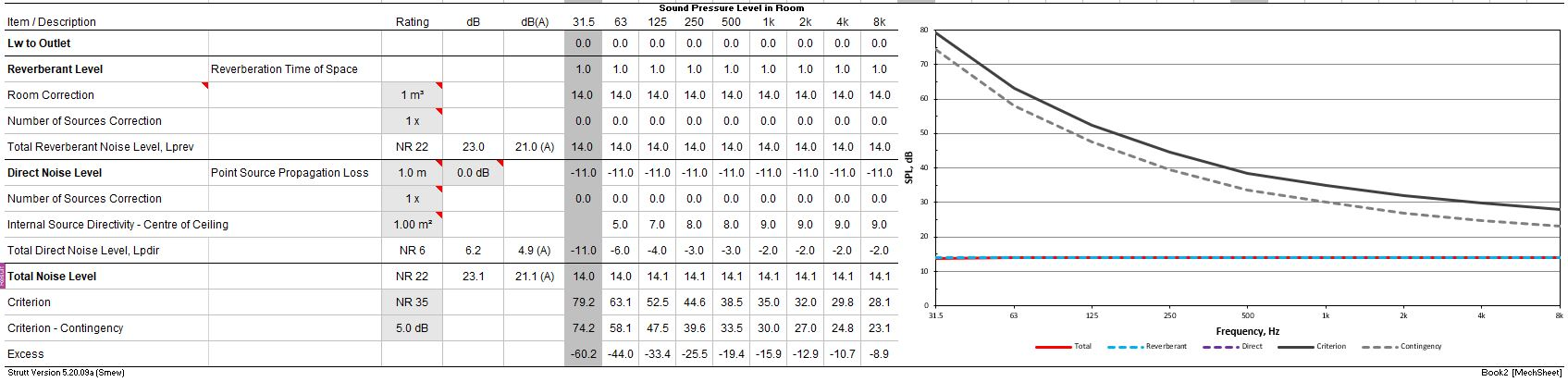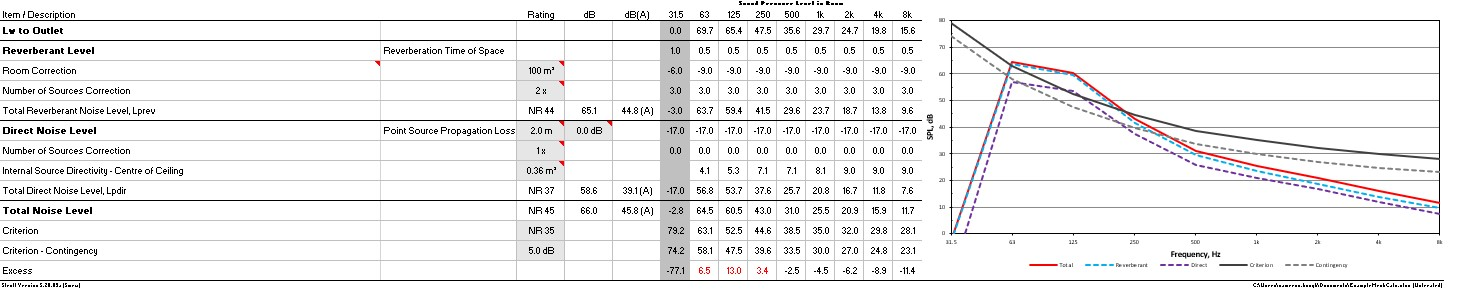﻿ Introduction to Mechanical Calculations### How To "Strutt" Series

Introduction to Mechanical Calculations    1/1, 1/3

This guide is intended as an introduction to mechanical calculations for those who are new to Strutt.

Strutt provides a series of formatted calculations sheets (with particular frequencies always located in particular columns), in addition to a ‘toolbox’ of functions/calculations. This allows the user to build up a calculation element by element.

A calculation ca be built in several different ways; the following is a recommendation of one such way to build a mechanical calculation in Strutt:

• Use the Mechanical sheet (the easiest way to insert Strutt sheets is to right-click on the ‘ply’ – the bar at the bottom of Excel with the sheet names; Strutt has a context-specific menu here. Sheets can also be added via the Strutt | Blank Sheets drop-down on the ribbon).
• Strutt has two tabs in the Excel ribbon: the Strutt tab has the full set of calculations, while Strutt Mech includes the most commonly used calculations for mechanical calculations.
• Work linearly down the duct from the sound power source to the room. For supply air, this is in the direction of airflow. For return air, start at the end of the air path and work back to the room.
• The mechanical sheet has three sets of columns:
• Insertion loss / attenuation – elements that reduce the sound level in the duct by a constant amount go here (e.g. the loss through a lined bend).
• In-duct sound power / regenerated noise – sound power that is introduced to the duct (e.g. via regenerated noise or duct break-in).
• Cumulative sound power – this keeps a running tally of the sound power level within the duct at each row. It’s calculated as the sound power of the previous row, added to the losses in the current row, and then logarithmically added to the sound power created in the current row.• Once all of the elements along the path have been added, you will have calculated a prediction of the final sound power at the end of the duct. The Mechanical sheet then has a section in which the room sound is calculated.• In order to calculate the reverberant and direct components of the sound, enter the following into this section of the sheet:
• room volume;
• reverberation time of the room (if you’re unsure of the reverberation time, the Quick RT function has typical ranges for different types of spaces);
• number of grilles in the room;
• distance from the grille to the receiver point and;
• grille area.
• Note that once calculations have been entered in any Strutt sheet (for example, the Internal Source Directivity – Centre of Ceiling row in the figure above), the parameters can be edited in the calculation by right-clicking and selecting Update Row from the context menu. This will open up the calculation userform, allowing the parameters to be updated.
• Commonly-modified values (e.g. grille area in the given example) are placed in the worksheet so that they can be edited on the fly without having to open the userform.
• The final row of the calculation – Excess – shows the difference between the calculated noise level and the design curve (e.g. the NR35 curve in the above example). This difference is a good first estimate of the insertion loss requirement for an attenuator (although sometimes a little trial and error is needed because other sound power sources, such as regenerated noise from VAV boxes or grilles, begins to dominate the noise level in the room; in this case, the noise level in the room isn’t solely due to the fan).

The example below shows that, after inserting the duct elements of a hypothetical duct run, there is an exceedance of the design curve at low frequencies. This excess curve then gives a good first estimate of the amount of attenuation required.Please see the help pages for each function for more details on the range of applicability and the requirements for input parameters (e.g. some of the duct attenuation calculations are only valid for ducts within a particular size or aspect ratio range).

Happy Strutting!